# A Million Bits of the Center Column of the Rule 30 Cellular Automaton

The center column of the rule 30 cellular automaton over a million steps of evolution

Originator: Stephen Wolfram

This data was presented in a graphic on page 871 of Stephen Wolfram's "A New Kind of Science".

(1000000 elements)

## Examples

### Basic Examples

Retrieve the resource:

Retrieve the default content:

 In:=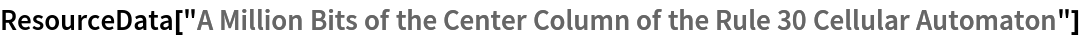Out=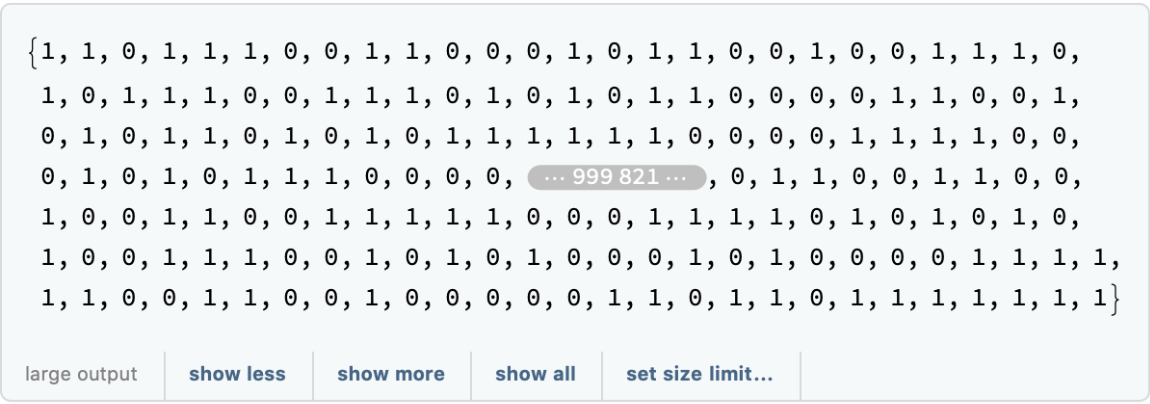Count the number of 1s and 0s:

 In:=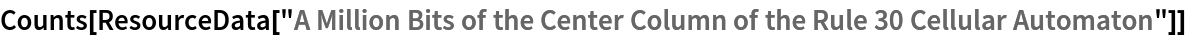Out=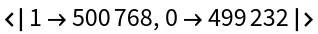Make a “random walk plot” of the first 100,000 elements:

 In:=Out=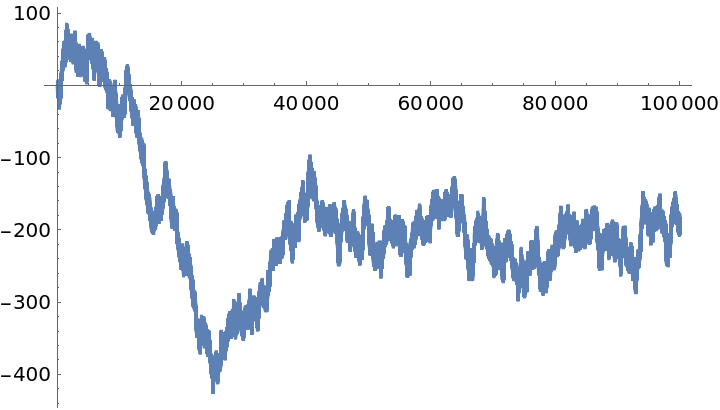Plot the distribution of displacements for the walk:

 In:=Out=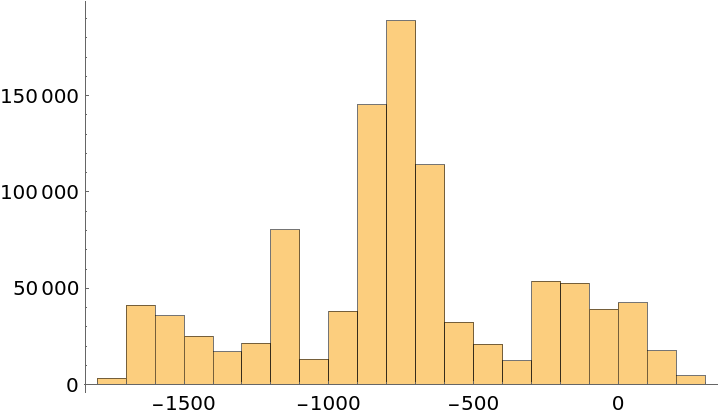Find the longest run of identical elements in the sequence:

 In:=Out=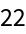Wolfram Research, "A Million Bits of the Center Column of the Rule 30 Cellular Automaton" from the Wolfram Data Repository (2017) https://doi.org/10.24097/wolfram.25316.data